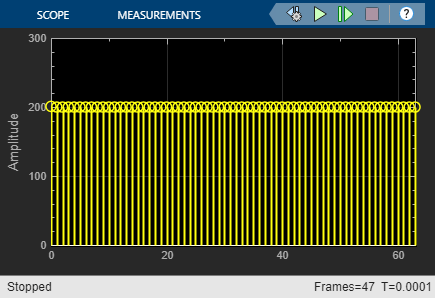This example shows how to model a 6-bit Subranging ADC with pipelining and an error correcting second stage.

Subranging ADCs are typically faster than sigma delta and successive approximation ADCs but provide less resolution. Typical sample rates are in the 10M sample/sec range, and typical resolution is 8 to 16 bits. Additional stages and/or bits per stage yield higher resolutions.

The subranging ADC is a two stage data converter. The first stage converter is a 3-bit Flash ADC. It drives a 3-bit ideal DAC, which in turn drives the second stage. The second stage converter is 4-bit flash ADC. The extra bit corrects the errors in the first ADC and improves conversion accuracy. The sampling rate of the ADC is `100` MHz, and is defined in the model initialization callback by MATLAB® variable Fs. Additional MATLAB® variables N1, N2, and NBits set the number of bits for the first stage, second stage and overall converter respectively.

The model is based on the following Analog Devices tutorial: ADC Architectures V: Pipelined Subranging ADCs.

The first ADC serves as a coarse 3 bit converter. The quantization error due to the first ADC is itself quantized by the second ADC. To generate this error signal, a 3-bit DAC converts the coarse ADC output to an analog signal which is subtracted against the original analog input. The difference is the residue signal. The residue signal is amplified and converted back to a digital signal by the second ADC. The 3 bit ADC output (MSBs) and the 4-bit ADC output (LSBs) are concatenated to form an overall 6 bit unsigned ADC output.

```model = 'subranging_adc_ac'; load_system(model); sc = get_param([model '/Spectrum Analyzer'], 'ScopeConfiguration'); sc.OpenAtSimulationStart = false; open_system(model); ```### Dynamic Testing

This model uses one of two test sources. Use a sine wave for dynamic testing, e.g. ENoB, SNR, and SFDR. Use a ramp signal for static testing, e.g. missing codes and nonlinearity quantifiers.

To determine SNR, ENOB and other dynamic characteristics of the subranging ADC, use the ADC AC Measurement block from the Mixed-Signal Blockset™. Select the correct switch position to use the Sine Wave as input source. Set the simulation stop time using the button on the mask of the ADC AC Measurement block and set its Distortion measurement type is set to `Harmonic`.

```set_param([model '/Source Select'],'sw','1'); set_param([model '/ADC AC Measurement'], 'InputFrequency', '10e6'); open_system([model '/Time Scope']); sim(model); ```Add another sine wave to the input to test intermodulation distortion. Observe and measure the results using the ADC AC Measurement block with its Distortion measurement type set to `Intermodulation`.

```set_param([model '/Source Select'],'sw','0'); set_param([model '/ADC AC Measurement'], 'DistortionMeasurement', 'Intermodulation'); set_param([model '/ADC AC Measurement'], 'InputFrequency', '[9, 11] * 1e6'); sim(model); ```### Missing Code Analysis

This example uses a histogram block to plot the frequency of occurrence of each ADC output code. Use the Sawtooth Generator block as the input source by setting the input switch to the proper position.

```close_system(model, 0); model = 'subranging_adc_dc'; load_system(model); set_param([model '/MSB ADC'],'OffsetError','0'); set_param([model '/MSB ADC'],'GainError','0'); set_param([model '/Source Select'],'sw','1'); open_system(model); ```Set the simulation time to `1.28e-4` s, which is 10x longer than the recomended minimum simulation stop time from the ADC DC Measurement block. Given our sawtooth stimulus, this will cause our entire transfer curve to be swept 10 times, increasing the accuracy of the histogram by reducing the influence of random impairments such as aperture jitter.

```set_param(model, 'StopTime', '1.28e-4'); open_system([model '/ADC Output Histogram']); sim(model); ```You can observe how the second stage corrects errors from the first by adding Offset error and Gain error in the impairments tab of the MSB ADC. Any fraction of an LSB introduced as a form of gain or offset error results in an imbalance or missing code in the ADC output histogram. Anything other than a flat histogram with a sawtooth input signifies some amount of non-ideal ADC behavior in the form of integral non-linearity, differential non-linearity, or missing codes.

Total errors `max(abs(Offset error + Gain error, Offset error))` of up to 0.5 LSB in the MSB ADC are corrected by the extra bit of the LSB ADC. Larger errors in the MSB ADC and any errors in the LSB ADC influence the output.

```set_param([model '/MSB ADC'], 'OffsetError', '0.2'); set_param([model '/MSB ADC'], 'GainError', '0.2'); sim(model); ```View the results of the ADC DC Measurement block by clicking the Plot measurement results button in the block mask.```close_system(model, 0); model = 'subranging_adc_testbench'; load_system(model); sim(model); open_system(model); ```